# JP6538609B2 - Motion vector detection device, motion vector detection method and motion vector detection program - Google Patents

Motion vector detection device, motion vector detection method and motion vector detection program Download PDF

## Info

Publication number
JP6538609B2
JP6538609B2 JP2016095646A JP2016095646A JP6538609B2 JP 6538609 B2 JP6538609 B2 JP 6538609B2 JP 2016095646 A JP2016095646 A JP 2016095646A JP 2016095646 A JP2016095646 A JP 2016095646A JP 6538609 B2 JP6538609 B2 JP 6538609B2
Authority
JP
Japan
Prior art keywords
search
motion vector
frame
detected
block
Prior art date
Legal status (The legal status is an assumption and is not a legal conclusion. Google has not performed a legal analysis and makes no representation as to the accuracy of the status listed.)
Active
Application number
JP2016095646A
Other languages
Japanese (ja)
Other versions
JP2017204752A (en
Inventor

Original Assignee

Priority date (The priority date is an assumption and is not a legal conclusion. Google has not performed a legal analysis and makes no representation as to the accuracy of the date listed.)
Filing date
Publication date
Application filed by 日本電信電話株式会社 filed Critical 日本電信電話株式会社
Priority to JP2016095646A priority Critical patent/JP6538609B2/en
Publication of JP2017204752A publication Critical patent/JP2017204752A/en
Application granted granted Critical
Publication of JP6538609B2 publication Critical patent/JP6538609B2/en
Application status is Active legal-status Critical
Anticipated expiration legal-status Critical

• 238000001514 detection method Methods 0 claims description title 9
• 238000004364 calculation methods Methods 0 description 113
• 230000015654 memory Effects 0 description 35
• 238000000034 methods Methods 0 description 19
• 230000001131 transforming Effects 0 description 17
• 230000002829 reduced Effects 0 description 11
• 230000001629 suppression Effects 0 description 11
• 239000002609 media Substances 0 description 9
• 238000003860 storage Methods 0 description 6
• 230000000694 effects Effects 0 description 5
• 230000001976 improved Effects 0 description 4
• 239000010410 layers Substances 0 description 4
• 230000001603 reducing Effects 0 description 3
• 238000006722 reduction reaction Methods 0 description 3
• 238000004891 communication Methods 0 description 2
• 230000001276 controlling effects Effects 0 description 2
• 230000004044 response Effects 0 description 2
• 230000000875 corresponding Effects 0 description 1
• 239000000203 mixtures Substances 0 description 1
• 230000002093 peripheral Effects 0 description 1
• 239000004065 semiconductor Substances 0 description 1
• 239000003826 tablets Substances 0 description 1
• 230000002123 temporal effects Effects 0 description 1

## Images

•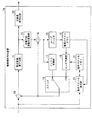•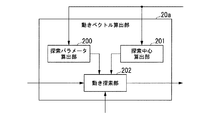•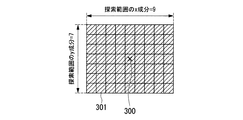••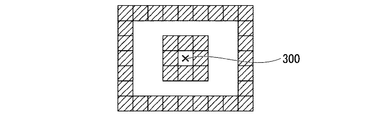•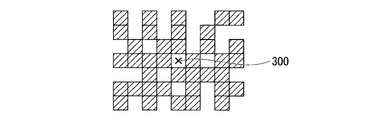•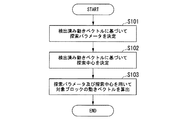•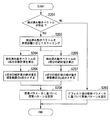•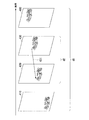•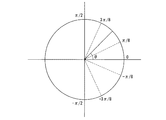•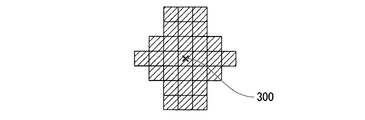••••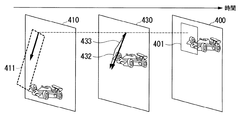•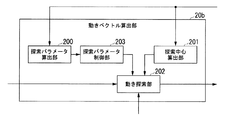•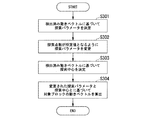•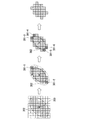••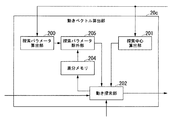••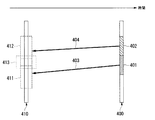•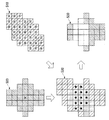•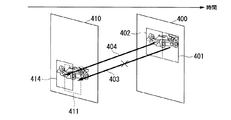•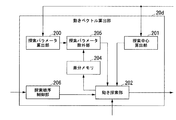•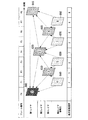•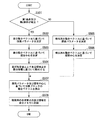•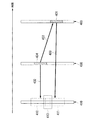••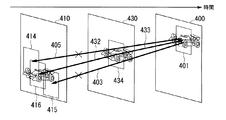•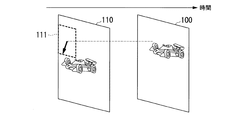•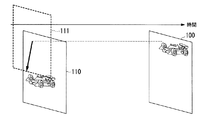•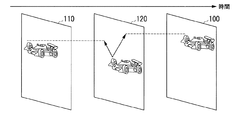••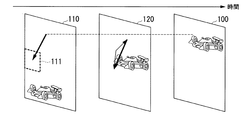## Description

The present invention relates to a motion vector detection device, a motion vector detection method, and a motion vector detection program.

Moving picture coding standards such as H.264 / AVC and H.265 / HEVC (High Efficiency Video Coding) have been formulated as moving picture coding standards. In video coding, a frame is divided into a plurality of blocks. In video coding, the amount of information of a coded video can be reduced by switching between intraframe prediction coding and interframe prediction coding for each block. Intra-frame prediction coding is coding that predicts a motion vector using information in a frame. Inter-frame predictive coding is coding that predicts a motion vector using coded frame information between frames.

In interframe predictive coding, a differential image is calculated by using a reference frame (reference image) as a predictive image. The reference frame is a frame that is referenced from the target frame of encoding using a motion vector. The amount of information of a coded moving image is reduced by coding using a motion vector and a difference image.

The motion vector is calculated by motion search (motion vector search). Motion search is to search a region most similar to a block in a first frame such as a target frame in a second frame such as a reference frame. In the motion search, for example, a motion vector directed from a target block in a target frame to a region identified in the reference frame is calculated. The target block in the target frame is efficiently encoded if an accurate motion vector is calculated. As a general problem in motion search, there is a problem regarding a search range.

FIG. 31 is a diagram illustrating an example of the search range 111 when the reference distance is short. FIG. 32 is a diagram showing an example of the search range 111 when the reference distance is long. The search range 111 is expanded as the temporal distance (reference distance) between the target frame 100 and the reference frame 110 is longer. The amount of operation for encoding increases as the search range 111 is expanded. Therefore, in inter-frame predictive coding, a method for efficiently calculating a motion vector with a small amount of operation is desired. Non-Patent Document 1 discloses a method for efficiently calculating a motion vector with a small amount of operation.

FIG. 33 is a diagram showing an example of a method of searching around the relay frame 120. As shown in FIG. The relay frame 120 is a frame located between the target frame 100 and the reference frame 110 in the time axis direction. A motion vector that refers to the target frame 100 from the relay frame 120 is calculated by performing a motion search centered on the relay frame 120. A motion vector that references the reference frame 110 from the relay frame 120 is also calculated by performing a motion search centered on the relay frame 120.

FIG. 34 is a diagram illustrating an example of a method of searching around a point at which motion vectors are connected. A motion vector referencing the target frame 100 from the relay frame 120 and a motion vector referencing the reference frame 110 from the relay frame 120 may be concatenated. The resulting resulting motion vectors are projected to the reference frame. The position indicated by the projected motion vector is defined as the center (search center) of the search in the reference frame. Since the motion search is started from a position close to the image of the object in the reference frame, the motion vector is accurately calculated even if the search range is not expanded.

JP, 2015-226085, A

FIG. 35 is a diagram illustrating an example in which the coding efficiency is reduced. When the object is moving while accelerating in the moving image, the search range can not be accurately defined. The motion vector can not be accurately calculated if the search range can not be accurately determined. That is, the conventional moving picture coding apparatus has a problem that the motion vector can not be efficiently calculated when the increase in the operation amount of the motion search is suppressed.

In view of the above circumstances, the present invention provides a motion vector detection device, a motion vector detection method, and a motion vector detection program capable of efficiently calculating a motion vector even if an increase in the amount of operation of motion search is suppressed. The purpose is that.

One embodiment of the present invention is a range determination unit that determines a length of a search range of a motion vector based on at least one of a statistic of a horizontal component and a statistic of a vertical component of a detected motion vector between frames; And a motion search unit for searching for the motion vector with respect to the search point in the search range for which is determined.

One embodiment of the present invention is the motion vector detection device described above, wherein the range determination unit determines the length of the search range according to a ratio between the statistic of the horizontal component and the statistic of the vertical component. And determine the shape of the search range based on the direction of the detected motion vector.

One embodiment of the present invention is the above-described motion vector detection device, wherein the range determination unit changes the length of the search range until the number of the search points in the search range becomes a predetermined value.

One embodiment of the present invention is the above-described motion vector detection device, wherein the range determination unit sets the change amount of the area of the search range within a threshold before and after changing the length of the search range.

One embodiment of the present invention is the motion vector detection device described above, wherein the detected motion vector is a motion vector that refers to a reference frame of a target block to be encoded from a target frame to which the target block belongs, The determination unit excludes, from the search range, the search point whose evaluation value at the time of detecting the first detected motion vector is equal to or less than a threshold.

One embodiment of the present invention is the motion vector detection device described above, wherein the detected motion vector is a first detected motion vector that refers to a reference frame of a target block to be encoded from a frame, and the target block And the range determination unit excludes, from the search range, the search point whose evaluation value at the time of detecting the detected motion vector is equal to or greater than a threshold value. Do.

One aspect of the present invention is a motion vector detection method executed by a motion vector detection device, which detects motion vectors based on at least one of statistics of horizontal components and statistics of vertical components of detected motion vectors between frames. A motion vector detection method comprising: determining a length of a search range; and searching for the motion vector for a search point in the search range whose length is determined.

One aspect of the present invention is a motion vector detection program for causing a computer to function as the above-described motion vector detection device.

According to the present invention, it is possible to efficiently calculate a motion vector even if the increase in the amount of operation of motion search is suppressed.

It is a figure which shows the example of a structure of a moving image encoding device in 1st Embodiment. It is a figure which shows the example of a structure of a motion vector calculation part in 1st Embodiment. It is a figure which shows the example of the search range in 1st Embodiment. It is a figure which shows the example of an elliptical search pattern in 1st Embodiment. It is a figure which shows the example of a donut shaped search pattern in 1st Embodiment. It is a figure which shows the example of a random type | mold search pattern in 1st Embodiment. 7 is a flowchart showing an example of the operation of the motion vector calculation unit 20 in the first embodiment. It is a flowchart which shows the example of the operation | movement for determining a search parameter in 1st Embodiment. It is a figure which shows the example of scaling of a detected motion vector in 1st Embodiment. It is a figure which shows the example of the direction of the vector which has a statistics value of x component, and a statistics value of y component in a 1st embodiment. It is a figure which shows the example of the 1st search pattern in 1st Embodiment. It is a figure which shows the example of the 2nd search pattern in 1st Embodiment. It is a figure which shows the example of the 3rd search pattern in 1st Embodiment. It is a figure which shows the example of the 4th search pattern in 1st Embodiment. It is a figure which shows the example of the method for determining a search pattern in 1st Embodiment. It is a figure which shows the example of a structure of a motion vector calculation part in 2nd Embodiment. It is a flowchart which shows the example of operation | movement of a motion vector calculation part in 2nd Embodiment. It is a figure which shows the example of a change of the search pattern by deleting a search point in 2nd Embodiment. It is a figure which shows the example of a change of the search pattern by adding a search point in 2nd Embodiment. It is a figure which shows the example of a structure of a motion vector calculation part in 3rd Embodiment. It is a flowchart which shows the example of operation | movement of a motion vector calculation part in 3rd Embodiment. It is a figure which shows the example of an object frame and a reference frame in 3rd Embodiment. It is a figure which shows the example of the method for excluding the search point with a small difference of the block of an object frame, and a reference block in 3rd Embodiment. It is a figure which shows the example of the effect by having excluded the search point with a small difference of the block of an object frame, and a reference block in 3rd Embodiment. It is a figure which shows the example of a structure of a motion vector calculation part in 4th Embodiment. It is a figure which shows the example of a motion search order in 4th Embodiment. It is a flowchart which shows the example of operation | movement of a motion vector calculation part in 4th Embodiment. It is a figure which shows the example of an object frame, a reference frame, and a motion vector detected frame in a 4th embodiment. It is a figure which shows the example of the method for excluding the search point with a large difference of the block of reference, and the block of flame | frames other than an object frame in 4th Embodiment. It is a figure which shows the example of the effect by having excluded the search point with a large difference of the block of reference, and the block of flame | frames other than an object frame in 4th Embodiment. It is a figure which shows the example of the search range in case reference distance is short. It is a figure which shows the example of the search range in case reference distance is long. It is a figure which shows the example of the method of searching centering on a relay frame. It is a figure which shows the example of the method of searching centering on the point which connected motion vector. It is a figure which shows the example to which encoding efficiency fell.

Embodiments of the present invention will be described in detail with reference to the drawings.
The moving picture coding apparatus according to the embodiment detects a motion vector of a target block in a target frame based on a target picture of a target frame to be coded, a search parameter, and a search center. Here, the motion vector is represented by a two-dimensional vector having components in the x direction (horizontal direction) and the y direction (vertical direction).

The video encoding apparatus of the embodiment determines a search parameter based on the detected motion vector. Here, the detected motion vector is a motion vector calculated before the motion vector of the target block in the target frame is detected. The detected motion vector may be a motion vector of a block of a frame whose motion vector has been detected among frames different from the target frame. The search parameter is a parameter that represents the shape of the search range (hereinafter referred to as “search pattern”). The search parameter is expressed, for example, using a length (value) in the x direction and a length (value) in the y direction. The search range includes one or more search points. The video encoding apparatus of the embodiment determines the search center based on the detected motion vector.

The moving picture coding apparatus according to the embodiment adaptively changes the search parameter based on the statistics of the detected motion vector. That is, the moving picture coding apparatus according to the embodiment changes the lengths in the x direction and y direction of the search range based on the x component (horizontal component) and the y component (vertical component) of the motion vector. Thus, the moving picture coding apparatus according to the embodiment can efficiently calculate the motion vector even if the increase in the amount of operation of the motion search is suppressed.

The video encoding apparatus of the embodiment may determine the search pattern based on the angle determined by the statistics of the detected motion vector. The moving picture coding apparatus according to the embodiment determines a search pattern based on a motion vector referring to a target block in a target frame. As a result, the moving picture coding apparatus according to the embodiment can efficiently search for a motion vector by defining a search range having a high probability that an optimal motion vector exists.

Hereinafter, the standard to which the video encoding device conforms is not limited to a specific standard. Hereinafter, as an example, a moving picture coding apparatus compliant with H. 265 / HEVC will be described.

First Embodiment
FIG. 1 is a diagram showing an example of the configuration of the video encoding device 1. The video encoding device 1 is an information processing terminal such as a personal computer device, a tablet terminal, a smartphone terminal, and a server device. The video encoding device 1 acquires a video to be encoded. The moving picture coding apparatus 1 acquires a target picture of a target frame of coding for each target block of coding. The target picture is image information in a target block of a target frame of encoding. The image information is, for example, information representing an image feature amount. The video encoding device 1 encodes a target block in raster scan order. The moving picture coding apparatus 1 transmits coded data representing the result of coding of a target block.

The moving picture coding apparatus 1 includes a subtractor 10, an orthogonal transformation / quantization unit 11, a variable length encoding unit 12, an inverse quantization / inverse orthogonal transformation unit 13, an adder 14, and a loop filter 15 A decoded picture memory 16, an intra prediction unit 17, an inter prediction unit 18, a switch 19, a motion vector calculation unit 20a, and a motion vector memory 21 are provided.

Subtractor 10, orthogonal transform / quantization unit 11, variable length coding unit 12, inverse quantization / inverse orthogonal transform unit 13, adder 14, loop filter 15, intra prediction unit 17, inter prediction unit 18, switch 19, and Part or all of the motion vector calculation unit 20a is a software function unit that functions when, for example, a processor such as a CPU (Central Processing Unit) executes a program stored in the memory. Further, some or all of these functional units may be hardware functional units such as LSI (Large Scale Integration) or ASIC (Application Specific Integrated Circuit).

The decoded picture memory 16 and the motion vector memory 21 are configured using a storage device having a non-volatile storage medium (non-temporary storage medium) such as a magnetic hard disk drive or a semiconductor storage device. The decoded picture memory 16 and the motion vector memory 21 may have, for example, a volatile storage medium such as a random access memory (RAM) or a register.

The subtractor 10 acquires a target block. The subtractor 10 acquires a predicted image from the intra prediction unit 17 or the inter prediction unit 18 via the switch 19. The subtractor 10 transmits a difference image, which is the difference between the target block and the predicted image, to the orthogonal transformation / quantization unit 11.

The orthogonal transformation / quantization unit 11 applies orthogonal transformation processing and quantization processing to the difference image. The orthogonal transformation / quantization unit 11 transmits the differential image subjected to the orthogonal transformation processing and the quantization processing to the variable-length coding unit 12 and the inverse quantization / inverse orthogonal transformation unit 13.

The variable-length coding unit 12 performs variable-length coding processing on the quantization coefficient transmitted from the orthogonal transform / quantization unit 11. The variable-length coding unit 12 transmits coded data representing the result of the coding process to the outside of the moving picture coding apparatus 1.

The inverse quantization / inverse orthogonal transformation unit 13 performs inverse quantization processing and inverse orthogonal transformation processing on the quantization coefficient transmitted from the orthogonal transformation / quantization unit 11. The inverse quantization / inverse orthogonal transform unit 13 transmits an image representing the result of the inverse quantization process and the inverse orthogonal transform process to the adder 14.

The adder 14 obtains an image representing the result of the inverse quantization process and the inverse orthogonal transformation process from the inverse quantization / inverse orthogonal transformation unit 13. The adder 14 obtains a predicted image from the subtractor 10. The adder 14 transmits the sum of the image transmitted from the inverse quantization / inverse orthogonal transform unit 13 and the prediction image to the intra prediction unit 17 and the loop filter 15.

The loop filter 15 acquires the prediction image from the intra prediction unit 17 or the inter prediction unit 18 via the adder 14. The loop filter 15 applies the loop filter to the sum of the image transmitted from the inverse quantization / inverse orthogonal transform unit 13 and the predicted image. The loop filter 15 records an image (decoded picture) representing the result of applying the loop filter in the decoded picture memory 16.

The decoded picture memory 16 stores an image representing the result of applying the loop filter. The image representing the result of applying the loop filter is used as the reference frame in the inter prediction unit 18 when the inter prediction unit 18 executes the inter prediction process of the target block.

The intra prediction unit 17 (intra-frame prediction unit) acquires an image representing the result of the inverse quantization process and the inverse orthogonal transformation process from the inverse quantization / inverse orthogonal transformation unit 13 via the adder 14. The intra prediction unit 17 acquires the predicted image transmitted by the intra prediction unit 17 via the adder 14. That is, the intra prediction unit 17 obtains, via the adder 14, the sum of the image transmitted from the inverse quantization / inverse orthogonal transform unit 13 and the prediction image transmitted by the intra prediction unit 17.

The intra prediction unit 17 may acquire the prediction image transmitted by the inter prediction unit 18 via the adder 14. That is, the intra prediction unit 17 may obtain the sum of the image transmitted from the inverse quantization / inverse orthogonal transform unit 13 and the prediction image transmitted by the inter prediction unit 18 via the adder 14. The intra prediction unit 17 generates an intra prediction image (intra-frame prediction image) of the target block based on the reference frame.

The inter prediction unit 18 (inter-frame prediction unit) acquires, from the decoded picture memory 16, a reference frame that is an image representing the result of applying the loop filter. The inter prediction unit 18 acquires a motion vector from the motion vector memory 21. The inter prediction unit 18 generates an inter prediction image (interframe prediction image) of the target block based on the reference frame and the motion vector.

The switch 19 acquires a prediction image from the intra prediction unit 17 or the inter prediction unit 18 according to the prediction mode determined for the target block. The switch 19 transmits the acquired predicted image to the subtractor 10 and the adder 14.

The motion vector calculation unit 20a (motion vector detection device) acquires a target block to be encoded. The motion vector calculation unit 20 a acquires a reference frame from the decoded picture memory 16. The motion vector calculation unit 20 a acquires the detected motion vector from the motion vector memory 21. The motion vector calculation unit 20a calculates a motion vector of the target block based on the target block, the reference frame, and the detected motion vector. The motion vector calculation unit 20 a records the motion vector of the target block in the motion vector memory 21.

The motion vector memory 21 stores the motion vector of the target block. The motion vector memory 21 transmits the motion vector of the target block to the motion vector calculation unit 20 according to the access. The motion vector memory 21 transmits the motion vector of the target block to the inter prediction unit 18 according to the access.

FIG. 2 is a diagram showing an example of the configuration of the motion vector calculation unit 20a. The motion vector calculation unit 20 a includes a search parameter calculation unit 200, a search center calculation unit 201, and a motion search unit 202. The search parameter calculation unit 200 determines a search parameter based on the detected motion vector. The search center calculation unit 201 determines a search center based on the detected motion vector.

The motion search unit 202 acquires a target picture of a target frame. The motion search unit 202 acquires a reference frame from the decoded picture memory 16. The motion search unit 202 acquires a search parameter from the search parameter calculation unit 200. The motion search unit 202 acquires a search center from the search center calculation unit 201. The motion search unit 202 performs a motion search process based on the target picture, the reference picture, the search parameter, and the search center. The motion search unit 202 detects the motion vector of the target block by motion search processing.

FIG. 3 is a diagram showing an example of a search range. The search center 300 is the center of the search range. In FIG. 3, the search pattern is rectangular. The x component of the search range is 9 as an example. That is, the number of search points 301 in the x direction of the search range is 9, as an example. The y component of the search range is 7 as an example. That is, the number of search points 301 in the y direction of the search range is 7, as an example.

FIG. 4 is a diagram showing an example of an elliptic search pattern. In FIG. 4, the search center 300 is the center of the elliptical search pattern (elliptic search area).
FIG. 5 is a diagram showing an example of a donut shaped search pattern. In FIG. 5, the search center 300 is the center of the donut-shaped search pattern (search area having a single empty area). In the donut search pattern, the search points are continuous.

FIG. 6 is a diagram showing an example of a random search pattern. The search points may not be arranged consecutively. For example, the search points may be randomly arranged. For example, the search pattern may not be vertically symmetrical. For example, the search pattern may not be symmetrical.

FIG. 7 is a flowchart showing an example of the operation of the motion vector calculation unit 20a. The search parameter calculation unit 200 (range determination unit) determines a search parameter based on the detected motion vector (step S101).

The search center calculation unit 201 determines the search center based on the detected motion vector. For example, the search center calculation unit 201 determines, as a search center, a point indicated by a detected motion vector that minimizes the difference between the target block of the target frame and the reference block of the reference frame. The difference between the target block and the reference block is represented, for example, by the sum of absolute values of differences. The difference between the target block and the reference block is represented by the sum of absolute values of differences in which the code amount of the motion vector is taken into consideration. If there is no detected motion vector, the search center calculation unit 201 may set the point indicated by the zero vector whose x component and y component are 0 as the search center (step S102).

The motion search unit 202 executes a motion search process on the target block based on the search parameter and the search center. Specifically, the motion search unit 202 calculates the difference between the target block and the reference block for the search center of the search range in the reference frame. The motion search unit 202 also calculates the difference (difference information) between the target block and the reference block for each search point other than the search center. The difference between the target block and the reference block is, for example, the difference between the image feature amount of the target block and the image feature amount of the reference block. The motion search unit 202 detects a motion vector indicating a search point at which the difference between the target block and the reference block is minimized.

FIG. 8 is a flowchart showing an example of an operation for determining a search parameter. The search parameter calculation unit 200 determines whether a detected motion vector exists (step S201). If the detected motion vector does not exist (step S201: NO), the search parameter calculation unit 200 determines a search pattern based on the search parameter of the default value (step S202). If there is a detected motion vector (step S201: YES), the search parameter calculation unit 200 performs scaling of the detected motion vector according to the reference distance. That is, the search parameter calculation unit 200 compresses or expands the length of the detected motion vector according to the reference distance (step S203).

FIG. 9 is a diagram illustrating an example of scaling of a detected motion vector. The reference frame 420 is a reference frame of the motion vector-detected frame 430. The motion vector detected frame 430 has a detected motion vector 431. The scaled detected motion vector is represented by equation (1).

mv_scale = mv × (d1 / d2) (1)

mv_scale indicates a scaled detected motion vector. That is, mv_scale indicates a detected motion vector which has been expanded or reduced. mv indicates a detected motion vector. d1 indicates the inter-frame distance between the target frame 400 and the reference frame 410. d2 indicates the inter-frame distance between the reference frame 420 and the motion vector-detected frame 430.

As shown in FIG. 8, the search parameter calculation unit 200 calculates the statistical value of the x component of the scaled detected motion vector (step S204). The search parameter calculation unit 200 calculates the statistical value of the y component of the scaled detected motion vector. The statistics of the motion vector are, for example, maximum value, minimum value, average value, total value, median value, variance value, and mode value. The statistical value of the motion vector may be, for example, a weighted sum of the maximum value, the minimum value, the average value, the total value, the median, the variance value, and the mode value (step S205).

The search parameter calculation unit 200 determines the statistical value of the scaled x component of the detected motion vector as the x component of the search range (step S206). The search parameter calculation unit 200 determines the statistical value of the y component of the scaled detected motion vector as the y component of the search range.

The search parameter calculation unit 200 determines a search pattern based on the search parameter (step S208).

(First example of search pattern determination)
FIG. 10 is a diagram showing an example of the direction of a vector having x-component statistics and y-component statistics as components. In the first example of search pattern determination, the search parameter calculation unit 200 is based on an angle θ formed by the statistic of the x component of the scaled detected motion vector and the statistic of the y component of the scaled detected motion vector. Determine the search pattern. That is, the search parameter calculation unit 200 determines the search pattern based on the direction of the vector having the statistical value of the x component and the statistical value of the y component as its components. For example, the search parameter calculation unit 200 determines the length of the search range in accordance with the ratio between the statistical value of the x component and the statistical value of the y component. The angle θ is expressed by equation (2).

θ = arctan (statistical value of y component / statistical value of x component) (2)

The angle θ is a value corresponding to the ratio between the statistical value of the x component and the statistical value of the y component. The angle θ indicates which proportion of the x component and the y component is higher. For example, when (−π / 4 ≦ θ <π / 4) holds, that is, when the ratio of the x component is higher than the y component, the search parameter calculation unit 200 selects a search pattern emphasizing the x direction. . For example, when (−π / 4 ≦ θ <π / 4) does not hold, that is, when the ratio of the y component is higher than the x component, the search parameter calculation unit 200 selects a search pattern in which the y direction is emphasized. .

FIG. 11 is a diagram showing an example of the first search pattern. When (−π / 2 ≦ θ <3π / 8) holds, the search parameter calculation unit 200 selects the first search pattern as the search pattern.

FIG. 12 is a diagram illustrating an example of a second search pattern. The search parameter calculation unit 200 selects the second search pattern as the search pattern when (-3π / 8 ≦ θ <−π / 8) holds.

FIG. 13 is a diagram illustrating an example of a third search pattern. When (−π / 8 ≦ θ <π / 8) is satisfied, the search parameter calculation unit 200 selects the third search pattern as the search pattern.

FIG. 14 is a diagram illustrating an example of a fourth search pattern. When (π / 8 ≦ θ <3π / 8) holds, the search parameter calculation unit 200 selects the fourth search pattern as the search pattern.

(Second example of search pattern determination)
In the second example of search pattern determination, the search parameter calculation unit 200 determines the search pattern based on the variance value (search parameter) of the detected motion vector. The search parameter calculation unit 200 calculates the variance of the detected motion vector. If the variance value of the detected motion vector is equal to or greater than the threshold value, it is considered that the motion of the image of the object in the frame has no rule in the sequence of the moving image. When the variance value of the detected motion vector is equal to or greater than the threshold, the search parameter calculation unit 200 selects a search pattern in which some search points are omitted. The search pattern in which some search points are omitted is, for example, a doughnut-shaped search pattern shown in FIG. The motion search unit 202 illustrated in FIG. 2 discretely searches for motion vectors using a search pattern in which some search points are omitted. Thus, the motion search unit 202 can efficiently calculate the motion vector even if the increase in the amount of operation of the motion search is suppressed.

(Third example of search pattern determination)
FIG. 15 is a diagram illustrating an example of a method for determining a search pattern. In the third example of search pattern determination, the search parameter calculation unit 200 determines a search pattern based on a detected motion vector that refers to a reference frame and a detected motion vector that refers to a target block.

The first detected motion vector 432 is a motion vector that refers to the reference frame 410 of the target block 401 of the target frame 400 in the motion vector detected frame 430. In the reference frame 410, a search range 411 is defined. The second detected motion vector 433 is a motion vector that refers to the target block 401 of the target frame 400 in the motion vector detected frame 430.

The first detected motion vector 432 is represented by equation (3). The second detected motion vector 433 is represented by equation (4).

mv1 = (mv1x, mv1y) (3)

mv2 = (mv2x, mv2y) (4)

mv1x is the x component of the first detected motion vector. mv1y is the y component of the first detected motion vector. mv2x is the x component of the second detected motion vector. mv2y is the y component of the second detected motion vector.

The search parameter calculation unit 200 searches for a search pattern based on the direction α (search parameter) of the difference vector between the first detected motion vector and the second detected motion vector (hereinafter referred to as “difference motion vector”). Determine The direction α of the differential motion vector is expressed by equation (5).

α = arctan ((mv2y−mv1y) / (mv2x−mv1x)) (5)

In FIG. 15, (π / 8 ≦ α <3π / 8) holds. Therefore, the direction α of the differential motion vector is biased obliquely to the upper right in FIG. In this case, the search parameter calculation unit 200 selects a search pattern biased in the upper right diagonal direction based on the direction of the difference motion vector (search parameter). The search pattern biased in the upper right diagonal direction is, for example, the fourth search pattern shown in FIG. Thus, the motion search unit 202 can efficiently calculate the motion vector even if the increase in the amount of operation of the motion search is suppressed.

As described above, the motion vector calculation unit 20 a (motion vector detection device) according to the first embodiment includes the search parameter calculation unit 200 and the motion search unit 202. The search parameter calculation unit 200 determines the length of the search range of the motion vector based on at least one of the statistics of the horizontal component and the statistics of the vertical component of the detected motion vector between frames. The motion search unit 202 searches for a motion vector for a search point in the search range whose length has been determined.

Thus, the moving picture coding apparatus 1 according to the first embodiment can efficiently calculate the motion vector even if the increase in the amount of operation of the motion search is suppressed. The moving picture coding apparatus 1 according to the first embodiment does not reduce the coding efficiency because the accuracy of the predicted picture is not reduced even when the motion vector is not accurately calculated because the object in the image is accelerated or the like.

That is, as in the first example of search pattern determination, the moving picture coding apparatus 1 of the first embodiment adaptively changes the search parameter based on the statistical value of the detected motion vector. Thus, the moving picture coding apparatus 1 according to the first embodiment can calculate an appropriate motion vector while suppressing an increase in the amount of operation of motion search.

The moving picture coding apparatus 1 according to the first embodiment adaptively changes the search parameter based on the angle formed by the statistics of the detected motion vector, as in the second example of search pattern determination. As a result, the moving picture coding apparatus 1 according to the first embodiment can calculate the motion vector efficiently by determining the range in which the probability that the optimal motion vector exists is high.

The moving picture coding apparatus 1 according to the first embodiment adaptively changes search parameters based on a motion vector referring to a target block, as in the third example of search pattern determination. As a result, the moving picture coding apparatus 1 according to the first embodiment can calculate the motion vector efficiently by determining the range in which the probability that the optimal motion vector exists is high.

Second Embodiment
In the second embodiment, the configuration and operation of the motion vector calculation unit are different from those in the first embodiment. In the second embodiment, only differences from the first embodiment will be described.

FIG. 16 is a diagram showing an example of the configuration of the motion vector calculation unit 20b. The motion vector calculation unit 20b of the video encoding device 1 includes a search parameter calculation unit 200, a search center calculation unit 201, and a motion search unit 202. The motion vector calculation unit 20 b further includes a search parameter control unit 203.

The search parameter control unit 203 changes the search parameter. That is, the search parameter control unit 203 changes the number of search points included in the search range of the search pattern determined by the search parameter. The search parameter control unit 203 adds or deletes a search point from the search range of the search pattern until the number of search points becomes a predetermined value. The predetermined value is a predetermined value. The search parameter control unit 203 transmits the changed search parameter to the motion search unit 202.

FIG. 17 is a flowchart illustrating an example of the operation of the motion vector calculation unit 20b. The search parameter calculation unit 200 determines search parameters based on the detected motion vector (step S301). The search parameter control unit 203 changes the search parameter until the number of search points included in the search range of the search pattern becomes a predetermined value (step S302). The search center calculation unit 201 determines a search center based on the detected motion vector (step S303). The motion search unit 202 calculates a motion vector of the target block based on the changed search parameter and the search center (step S304).

FIG. 18 is a diagram showing an example of change of a search pattern by deleting a search point. The search parameter control unit 203 acquires, from the search parameter calculation unit 200, a search parameter representing a fourth search pattern shown in FIG. 14 as an example. The search center 300 is the center of the fourth search pattern. The search parameter control unit 203 may acquire, from the search parameter calculation unit 200, search parameters representing a search pattern of an arbitrary shape such as a circle or a polygon.

The search parameter control unit 203 calculates an ellipse 302 of the smallest area that includes all the search points 301 included in the search range of the acquired search parameter. When the number of search points 301 included in the search range of the acquired search parameter is larger than a predetermined value, the search parameter control unit 203 maintains the elliptic shape of the ellipse 302 and keeps the ellipse 302 centered on the center 303. to shrink. The search parameter control unit 203 deletes the search point 301 until the number of search points 301 becomes a predetermined value. In FIG. 18, the search parameter control unit 203 deletes four search points 301. The search parameter control unit 203 preferentially deletes the search point 301 far from the center 303 among the search points 301 included in the ellipse 302, and deletes the search point 301. When there are a plurality of search points 301 at the same distance from the center 303, the search parameter control unit 203 preferentially deletes the search points 301 in which the raster scan order is first.

In FIG. 18, the predetermined value is 27, for example. In the initial state shown at the leftmost side of FIG. 18, the number of search points 301 is 33. The search parameter control unit 203 needs to delete six search points 301. The search parameter control unit 203 deletes the search points 301-1 and 301-2 outside the area of the reduced ellipse 302. The search parameter control unit 203 deletes the search points 301-3 to 301-6 outside the area of the further reduced ellipse 302.

FIG. 19 is a diagram showing an example of change of a search pattern by adding a search point. The search parameter control unit 203 acquires, from the search parameter calculation unit 200, a search parameter representing a fourth search pattern shown in FIG. 14 as an example. The center 303 is the center of the fourth search pattern. The search parameter control unit 203 may acquire, from the search parameter calculation unit 200, search parameters representing a search pattern of an arbitrary shape such as a circle or a polygon.

The search parameter control unit 203 calculates an ellipse 302. The ellipse 302 is an ellipse of the smallest area that includes all the search points 301 included in the search range of the search pattern determined by the search parameters acquired by the search parameter control unit 203.

When the number of search points 301 included in the search range of the acquired search parameter is smaller than a predetermined value, the search parameter control unit 203 maintains the elliptic shape of the ellipse 302 and keeps the ellipse 302 centered on the center 303. Expanding. The search parameter control unit 203 adds the search points 301 until the number of search points 301 becomes a predetermined value. In FIG. 19, the search parameter control unit 203 adds seven search points 301. The search parameter control unit 203 gives priority to the search point 301 closer to the center 303 among the search points 301 included in the ellipse 302, and adds the search point 301. When there are a plurality of search points 301 located at the same distance from the center 303, the search parameter control unit 203 preferentially adds the search points 301 whose raster scan order is first.

In FIG. 19, the predetermined value is 40 as an example. In the initial state shown at the leftmost side of FIG. 19, the number of search points 301 is 33. The search parameter control unit 203 needs to add seven search points 301. The search parameter control unit 203 adds search points 301-7 to 301-10 outside the area of the enlarged ellipse 302. The search parameter control unit 203 adds search points 301-1 to 301-13 outside the area of the enlarged ellipse 302. In FIG. 19, the search parameter control unit 203 does not add the search point 301-14. This is because the order of raster scanning of the search points 301-14 is later than the order of raster scanning of the search points 301-13.

When the search parameter control unit 203 adds or deletes a search point from the search range (changes the length of the search range) until the number of search points becomes a predetermined value, the search range is added by the search point. Or it may be the same area before and after being deleted. Therefore, the search parameter control unit 203 may not change the area of the search range even if the shape of the search range is changed before and after adding or deleting the search point. Further, the search parameter control unit 203 may store the change amount of the area of the search range within the threshold before and after adding or deleting the search point. Therefore, the change width of the predetermined value may be equal to or less than the threshold value so that the area of the search range becomes almost the same area before and after the search point is added or deleted.

As described above, the search parameter calculation unit 200 of the motion vector calculation unit 20b of the second embodiment changes the length of the search range until the number of search points in the search range becomes a predetermined value. The search parameter calculation unit 200 may store the change amount of the area of the search range within the threshold before and after changing the length of the search range.

As a result, the moving picture coding apparatus 1 of the second embodiment can efficiently calculate the motion vector even if the increase in the amount of operation of the motion search is suppressed. The moving picture coding apparatus 1 according to the second embodiment does not reduce the coding efficiency because the accuracy of the predicted picture is not reduced even when the motion vector is not accurately calculated because the object in the image is accelerated or the like.

That is, the moving picture coding apparatus 1 of the second embodiment changes the search parameter by adding or deleting the search points until the number of search points becomes a predetermined value. Thus, the moving picture coding apparatus 1 according to the second embodiment can calculate an appropriate motion vector by controlling the operation amount of the motion search. Since the amount of processing per unit time is fixed in hardware implementation, it is effective to control the amount of operation of motion search.

The moving picture coding apparatus 1 of the second embodiment expands or reduces the search range so that the search patterns have similar shapes before and after the expansion or reduction of the search range. By this, the moving picture coding apparatus 1 of the second embodiment improves the accuracy of the motion vector compared with the case of simply adding or deleting the search point, and efficiently controls the operation amount of the motion search. Can. The moving picture coding apparatus 1 of the second embodiment can efficiently control the amount of operation of motion search while maintaining the accuracy of the motion vector as compared with the case of simply adding or deleting a search point. .

As the moving picture coding apparatus 1 expands or reduces the search range of the search pattern, the following effects (1), (2) and (3) can be obtained.
(1) When the video encoding device 1 reduces the search range, the amount of operation of motion search decreases. The accuracy of the predicted image may decrease. The coding efficiency may be reduced.
(2) When the video encoding device 1 does not change the area of the search range (when the area of the search range before enlargement or reduction and the area of the search range after enlargement or reduction are substantially the same )
The amount of decrease or increase in the amount of operation of motion search is small. The accuracy of the predicted image is improved. The coding efficiency is improved.
(3) When the video encoding device 1 expands the search range,
The amount of operation of motion search increases. As compared with the above (2), the accuracy of the predicted image is further improved. The coding efficiency is further improved compared to the above (2).

Third Embodiment
The third embodiment is different from the first embodiment in the configuration and operation of a motion vector calculation unit. In the third embodiment, only differences from the first embodiment will be described.

FIG. 20 is a diagram showing an example of the configuration of the motion vector calculation unit 20c. The motion vector calculation unit 20c of the video encoding device 1 includes a search parameter calculation unit 200, a search center calculation unit 201, and a motion search unit 202. The motion vector calculation unit 20 c further includes a difference memory 204 and a search parameter exclusion unit 205. Hereinafter, a block whose motion vector has been detected is referred to as a “motion vector detected block”.

The motion search unit 202 records difference information (evaluation value) in the difference memory 204. The difference information is information representing the difference between the image feature amount of the first block and the image feature amount of the second block. The first block is, for example, the target block 401 of the target frame 400. The first block may be a block other than the target block 401 among the blocks of the target frame 400. For example, the first block may be a motion vector detected block of the target frame 400. The first block may be a block of a frame other than the target frame 400. The second block is, for example, a reference block of the reference frame 410.

The difference memory 204 acquires difference information (evaluation value) from the motion search unit 202. The difference memory 204 stores difference information. The difference memory 204 transmits difference information to the search parameter exclusion unit 205 in response to the access.

The search parameter exclusion unit 205 acquires a search parameter from the search parameter calculation unit 200. The search parameter exclusion unit 205 acquires difference information from the difference memory 204. The search parameter exclusion unit 205 excludes, from the search range of the acquired search parameters, search points for which the difference (difference information) between the block other than the target block and the reference block in the target frame is less than or equal to a threshold. The search parameter exclusion unit 205 transmits, to the motion search unit 202, search parameters representing the result of excluding the search point. When there is a possibility that all the search points are excluded from the search range, the search parameter exclusion unit 205 excludes from the search range the search point with the largest difference between the block other than the target block and the reference block in the target frame. You do not have to.

FIG. 21 is a flowchart showing an example of the operation of the motion vector calculation unit 20c. The search parameter calculation unit 200 detects a detected motion vector that refers to the same reference frame as the target block among the motion vectors of the motion vector detected block that belongs to the same target frame as the target block (hereinafter referred to as “the same frame reference motion vector” ) Is determined (step S401).

When the same frame reference motion vector exists (step S401: YES), the search parameter calculation unit 200 determines a search parameter based on the same frame reference motion vector (step S402). The search center calculation unit 201 determines the search center based on the same frame reference motion vector (step S403).

The search parameter exclusion unit 205 excludes a search point having a difference (difference information) between a block other than the target block and the reference block in the target frame that is equal to or less than a threshold from the search range of the acquired search parameter (step S404). The motion vector calculation unit 20c advances the process to step S407.

If the same frame reference motion vector does not exist (step S401: NO), the search parameter calculation unit 200 determines the search parameter based on the detected motion vectors other than the same frame reference motion vector (step S405). The search center calculation unit 201 determines the search center based on the detected motion vectors other than the same frame reference motion vector (step S406). The motion vector calculation unit 20c advances the process to step S407.

The motion search unit 202 detects a motion vector of the target block based on the search parameter and the search center (step S407). The motion search unit 202 records the difference information in the difference memory 204 (step S408).

FIG. 22 is a diagram showing an example of the target frame 400 and the reference frame 410. The target block 401 is a block to be encoded. The target block 401 belongs to the target frame 400. The motion vector detected block 402 is a block in which a motion vector has been detected. The motion vector detected block 402 belongs to the target frame 400. The search range 411 is a search range of the motion vector of the target block 401. The search range 412 is a search range of motion vectors of the motion vector detected block 402. The overlapping range 413 is a range in which the search range 411 and the search range 412 overlap.

The search center vector 403 is a motion vector of the target block 401, and is a motion vector indicating a search center of the search range 411 of the reference frame 410. The search center vector 404 is a motion vector of the motion vector detected block 402 and is a detected motion vector indicating the search center of the search range 412 of the reference frame 410. Therefore, the search center vector 404 is the same frame reference motion vector.

Since the same frame reference motion vector exists, the search parameter calculation unit 200 determines the search parameter based on the search center vector 404 which is the same frame reference motion vector. The search center calculation unit 201 determines a search center based on the search center vector 404.

FIG. 23 is a diagram illustrating an example of a method for excluding a search point having a small difference between the block of the target frame and the reference block. The search pattern 500 indicates a search pattern associated with the target block 401. The search pattern 510 shows a search pattern associated with the motion vector detected block 402. The value described in the search pattern 510 is a value representing the difference (difference information) between the motion vector detected block 402 and the reference block for each search point. In FIG. 23, the reference block is a block included in the search range 412 of the reference frame 410.

The search pattern 520 shows the result in which the search point of the search pattern 500 and the search point of the search pattern 510 are associated. That is, the search pattern 520 shows a result in which the search pattern 500 and the search pattern 510 are overlapped with the positions of the search points. Search points where the difference between the motion vector detected block 402 and the reference block is equal to or less than the threshold are indicated using black dots. The threshold is 50 as an example.

The search parameter exclusion unit 205 shown in FIG. 20 excludes, of the overlapping search points, search points having a difference between the motion vector detected block 402 and the reference block equal to or less than a threshold from the search range of the acquired search parameters. Do. In FIG. 23, the search parameter exclusion unit 205 excludes 11 search points. The search pattern 530 shows the search pattern of the changed search parameter. The search parameter exclusion unit 205 transmits a search parameter representing the search pattern 530 to the motion search unit 202.

As described above, the search center vector 404 which is the detected motion vector of the third embodiment is a motion vector that refers to the reference frame 410 of the current block 401 to be coded from the current frame 400 to which the current block 401 belongs. The search parameter calculation unit 200 of the motion vector calculation unit 20c according to the third embodiment searches for a search point whose difference information (evaluation value) at the time of detecting the search center vector 404 which is a detected motion vector is equal to or less than a threshold. Exclude from

Thus, the moving picture coding apparatus 1 according to the third embodiment can efficiently calculate the motion vector even if the increase in the amount of operation of the motion search is suppressed. The moving picture coding apparatus 1 according to the third embodiment does not reduce the coding efficiency because the accuracy of the predicted picture is not reduced even when the motion vector is not accurately calculated because the object in the image is accelerated or the like.

That is, the moving picture coding apparatus 1 of the third embodiment determines the destination pointed to by the motion vector of the target block 401 based on the difference information calculated when the search center vector 404 which is the same frame reference motion vector is calculated. Exclude search points that are unlikely to be The search point with low possibility of being pointed by the motion vector of the target block 401 is a search point with low probability that the target block becomes a candidate. Thus, the moving picture coding apparatus 1 according to the third embodiment can calculate an appropriate motion vector by controlling the operation amount of the motion search.

FIG. 24 is a diagram showing an example of an effect of excluding a search point having a small difference between the motion vector detected block 402 of the target frame 400 and the reference block 414. The reference block 414 is a block to which the motion vector detected block 402 refers. The possibility that different blocks in the target frame 400 overlap in the reference frame 410 is low. For example, the possibility that the target block 401 and the motion vector detected block 402 overlap in the reference frame 410 is low. Therefore, even if a search point (an area of an image similar to the image of the motion vector detected block 402) having a small difference from the motion vector detected block 402 is excluded, the target block 401 different from the motion vector detected block 402. The accuracy of the predicted image does not decrease.

Fourth Embodiment
The fourth embodiment is different from the first embodiment in the configuration and operation of a motion vector calculation unit. In the fourth embodiment, only differences from the first embodiment will be described.

FIG. 25 is a diagram showing an example of the configuration of the motion vector calculation unit 20 d. The motion vector calculation unit 20 d of the video encoding device 1 includes a search parameter calculation unit 200, a search center calculation unit 201, and a motion search unit 202. The motion vector calculation unit 20 d further includes a difference memory 204, a search parameter exclusion unit 205, and a search order control unit 206.

Hereinafter, the order of frames subjected to motion search is referred to as “motion search order”. The search order control unit 206 changes the motion search order in the order of the frames referenced in the encoding process based on the reference structure in the encoding process.

FIG. 26 is a diagram showing an example of the motion search order. The frame 600 and the frame 610 belong to the first layer. Frame 620 belongs to the second layer. The frames 630 and 660 belong to the third layer. The frames 640, 650, 670 and 680 belong to the fourth layer.

In FIG. 26, the video encoding apparatus 1 encodes the frame 600, the frame 610, the frame 620, the frame 640, the frame 650, the frame 660, the frame 670, and the frame 680 based on a hierarchical reference structure.

The frames referred to in the encoding process are, in the order of reference, the frame 640, the frame 650, the frame 670, the frame 680, the frame 630, the frame 660, the frame 620, and the frame 610. Therefore, the frame numbers of the frames referred to in the encoding process are F1, F3, F5, F7, F2, F6, F4, and F8 in the order of reference.

The motion search order is frame 640, frame 650, frame 670, frame 680, frame 630, frame 660, frame 620, frame 610, before being changed. The search order control unit 206 changes the motion search order to be in the order of the frames to be referred to based on the hierarchical reference structure. Thus, the motion search order is changed to frame 640, frame 650, frame 670, frame 680, frame 630, frame 660, frame 620, frame 610. The search order control unit 206 transmits the target pictures of the target frame to the motion search unit 202 in order of motion search.

The motion search unit 202 illustrated in FIG. 25 acquires the target pictures of the target frame from the search order control unit 206 in the order in which the motion search is performed. The motion search unit 202 performs a motion search process based on the target picture, the reference picture, the search parameter, and the search center. The motion search unit 202 detects the motion vector of the target block by motion search processing.

The difference memory 204 acquires, from the motion search unit 202, the difference information calculated at the time of motion search. The difference memory 204 stores difference information. The difference memory 204 transmits difference information to the search parameter exclusion unit 205 in response to the access.

The search parameter exclusion unit 205 acquires a search parameter from the search parameter calculation unit 200. The search parameter exclusion unit 205 acquires difference information from the difference memory 204. The search parameter exclusion unit 205 excludes, from the search range of the acquired search parameters, search points for which the difference (difference information) between the block in the frame other than the target frame and the target block and the reference block is equal to or greater than a threshold. The search parameter exclusion unit 205 transmits, to the motion search unit 202, search parameters representing the result of excluding the search point. When there is a possibility that all the search points are excluded from the search range, the search parameter exclusion unit 205 excludes, from the search range, the search point having the smallest difference between the block in the frame other than the target frame and the reference block. It does not have to be.

Hereinafter, a condition that a detected motion vector possessed by a block of a frame different from the target frame and a detected motion vector pointing to a block of a reference frame of the target block is referred to as a “first condition”.

Hereinafter, a first detected motion vector held by a block of a frame different from the target frame points to a block of a reference frame of the target frame, and a second detected motion vector further held by the block having the first detected motion vector The condition that “I” points to the target block of the target frame is referred to as a “second condition”.

FIG. 27 is a flowchart illustrating an example of the operation of the motion vector calculation unit 20d. The search parameter calculation unit 200 determines whether or not both the first condition and the second condition are satisfied (step S501).

If both the first condition and the second condition are satisfied (step S501: YES), the search parameter calculation unit 200 determines a search parameter based on the difference motion vector (step S502). The search center calculation unit 201 determines a search center based on the difference motion vector (step S503).

The search parameter exclusion unit 205 excludes, from the search range of the acquired search parameter, a search point for which the difference (difference information) between the block in the frame other than the target frame and the reference block is equal to or greater than the threshold (step S504). The motion vector calculation unit 20d advances the process to step S507.

If the first condition or the second condition is not satisfied (step S501: NO), the search parameter calculation unit 200 determines a search parameter based on the detected motion vector other than the difference motion vector (step S505). The search center calculation unit 201 determines the search center based on the detected motion vector other than the difference motion vector (step S506). The motion vector calculation unit 20d advances the process to step S507.

The motion search unit 202 detects a motion vector of the target block based on the search parameter and the search center (step S507). The motion search unit 202 records the difference information in the difference memory 204 (step S508).

FIG. 28 is a diagram illustrating an example of the target frame, the reference frame, and the motion vector-detected frame. The motion vector detected frame 430 is a frame located between the target frame 400 and the reference frame 410 in the time axis direction. Therefore, the motion vector detected frame 430 is a frame different from the target frame 400.

In FIG. 28, the first detected motion vector 432 is a detected motion vector possessed by the motion vector detected block 434 of the motion vector detected frame 430. The first detected motion vector 432 points to a block of the search range 412 of the reference frame 410 of the target frame 400.

In FIG. 28, the second detected motion vector 433 is the detected motion vector of the motion vector detected block 434. The second detected motion vector 433 points to the target block 401 of the target frame 400. The motion vector detected block 434 is a block having a first detected motion vector 432.

The motion vector detection completed block 434 determines, for the target block 401, whether both the first condition and the second condition are satisfied. In FIG. 28, the motion vector detected block 434 is a block of the motion vector detected frame 430. The first detected motion vector 432 is a detected motion vector that points to a block of the reference frame 410 of the target frame 400. Therefore, the first condition is satisfied. In FIG. 28, the first detected motion vector 432 points to a block of the reference frame 410 of the target frame 400. The second detected motion vector 433 points to the target block of the target frame 400. Therefore, the second condition also holds.

The search parameter calculation unit 200 shown in FIG. 25 calculates a difference motion vector between the first detected motion vector 432 and the second detected motion vector 433. The search parameter calculation unit 200 determines search parameters based on the difference motion vector. The search center calculation unit 201 shown in FIG. 25 determines a search center based on the difference motion vector.

FIG. 29 is a diagram illustrating an example of a method for excluding a search point in which the difference between a block of a frame other than the target frame and a reference block is large. The search pattern 500 indicates a search pattern associated with the target block 401. The search pattern 540 indicates the search pattern associated with the motion vector detected block 434 for the first detected motion vector 432. The value described in the search pattern 540 is a value that represents the difference (difference information) between the motion vector detected block 434 and the reference block for each search point. In FIG. 29, the reference block is a block included in the search range 412 of the reference frame 410.

The search pattern 550 shows the result in which the search point of the search pattern 500 and the search point of the search pattern 540 are associated. That is, the search pattern 550 shows the result in which the search pattern 500 and the search pattern 540 are overlapped with the position of the search point. The search points whose difference between the target block 401 and the reference block is equal to or less than the threshold are indicated by using black stars. The threshold is 50 as an example.

The search parameter exclusion unit 205 shown in FIG. 25 excludes, of the overlapping search points, the search points for which the difference between the motion vector detected block 434 and the reference block is equal to or less than the threshold from the search range of the acquired search parameters. Do. In FIG. 29, the search parameter exclusion unit 205 excludes seven search points. The search pattern 530 shows the search pattern of the changed search parameter. The search parameter exclusion unit 205 transmits a search parameter representing the search pattern 530 to the motion search unit 202.

As described above, the detected motion vector of the fourth embodiment includes the first detected motion vector 432 which refers to the reference frame 410 of the current block 401 to be encoded from the motion vector detected frame 430, and the current block 401. And a second detected motion vector 433 referenced from the motion vector detected frame 430. The search parameter calculation unit 200 excludes, from the search range, a search point whose difference information (evaluation value) at the time of detecting the first detected motion vector 432 is equal to or greater than a threshold.

Thus, the moving picture coding apparatus 1 according to the fourth embodiment can efficiently calculate the motion vector even if the increase in the amount of operation of the motion search is suppressed. The moving picture coding apparatus 1 according to the fourth embodiment does not reduce the coding efficiency because the accuracy of the predicted picture is not reduced even when the motion vector is not accurately calculated because the object in the image is accelerated or the like.

That is, the moving picture coding apparatus 1 of the fourth embodiment is based on the target block 401 indicated by the second detected motion vector 433 and the difference information calculated when the first detected motion vector 432 is calculated. Search points that are unlikely to be pointed to by the motion vector of the target block 401 are excluded. The search point with low possibility of being pointed by the motion vector of the target block 401 is a search point with low probability that the target block becomes a candidate. Thus, the moving picture coding apparatus 1 according to the fourth embodiment can control the amount of operation of motion search to calculate an appropriate motion vector.

FIG. 30 is a diagram illustrating an example of an effect of excluding a search point where the difference between a block of a frame other than the target frame and a reference block is large. The reference block 414 is one of the block candidates to which the target block 401 refers. The reference block 415 is one of the block candidates to which the target block 401 refers. The reference block 416 is a block to which the motion vector detected block 434 refers.

The possibility that the target block 401 refers to a block having a large difference from the motion vector detected block 434 in frames other than the target frame 400 is low. For example, the target block 401 is unlikely to refer to the reference block 414 or reference block 415 having a large difference from the motion vector detected block 434 in the reference frame 410.

The image of the block indicated by the first detected motion vector 432 and the image of the block indicated by the second detected motion vector 433 are likely to be images of the same object. The image of the motion vector detected block 434 and the image of the target block 401 are likely to be similar. Therefore, even if a search point (an area of an image not similar to the image of motion vector detected block 434) having a large difference from motion vector detected block 434 is excluded, the target block indicated by second detected motion vector 433 The accuracy of the 401 predicted image does not decrease.

At least a part of the moving picture coding apparatus and the motion vector detection apparatus in the above-described embodiment may be realized by a computer. In that case, a program for realizing this function may be recorded in a computer readable recording medium, and the program recorded in the recording medium may be read and executed by a computer system. Here, the “computer system” includes an OS and hardware such as peripheral devices. The term "computer-readable recording medium" refers to a storage medium such as a flexible disk, a magneto-optical disk, a ROM, a portable medium such as a ROM or a CD-ROM, or a hard disk built in a computer system. Furthermore, “computer-readable recording medium” dynamically holds a program for a short time, like a communication line in the case of transmitting a program via a network such as the Internet or a communication line such as a telephone line. It may also include one that holds a program for a certain period of time, such as volatile memory in a computer system that becomes a server or a client in that case. Further, the program may be for realizing a part of the functions described above, or may be realized in combination with the program already recorded in the computer system. It may be realized using a programmable logic device such as an FPGA (Field Programmable Gate Array).

The embodiment of the present invention has been described in detail with reference to the drawings. However, the specific configuration is not limited to this embodiment, and includes design and the like within the scope of the present invention.

The present invention is applicable to a moving picture coding apparatus for coding moving pictures.

DESCRIPTION OF SYMBOLS 1 ... Video coding apparatus, 10 ... Subtractor, 11 ... Orthogonal transformation and quantization part, 12 ... Variable-length coding part, 13 ... Inverse quantization and inverse orthogonal transformation part, 14 ... Adder, 15 ... Loop filter 16 Decoding picture memory 17 Intra prediction unit 18 Inter prediction unit 19 Switch 20a to 20d Motion vector calculation unit 21 Motion vector memory 100 Target frame 110 Reference frame 111 Search range, 120: relay frame, 200: search parameter calculation unit, 201: search center calculation unit, 202: motion search unit, 203: search parameter control unit, 204: difference memory, 205: search parameter exclusion unit, 206: search Order control unit, 300 ... search center, 301 ... search point, 302 ... ellipse, 303 ... center, 400 ... target frame, 401 ... target block, 4 2 motion vector detected block 403 search center vector 404 search center vector 405 search center vector 410 reference frame 411 search range 412 search range 413 overlapping range 414 reference block 415 ... reference block, 416 ... reference block, 420 ... reference frame, 430 ... motion vector detected frame, 431 ... detected motion vector, 432 ... first detected motion vector, 433 ... second detected motion vector 434: motion vector detected block 500: search pattern 510: search pattern 520: search pattern 530: search pattern 540: search pattern 550: search pattern 560: search pattern 600: frame 610 Frame, 620 ... Frame, 6 0 ... frame, 640 ... frame, 650 ... frame, 660 ... frame, 670 ... frame, 680 ... frame

## Claims (5)

1. A range determination unit that determines a length of a search range of a motion vector based on at least one of a statistic of a horizontal component and a statistic of a vertical component of a detected motion vector between frames;
A motion search unit for searching the motion vector for a search point in the search range for which the length is determined ;
The detected motion vector is a motion vector that refers to a reference frame of a current block to be encoded from a current frame to which the current block belongs,
The range determination unit excludes, from the search range, the search point whose evaluation value at the time of detecting the detected motion vector is equal to or less than a threshold.
Motion vector detection device.
2. A range determination unit that determines a length of a search range of a motion vector based on at least one of a statistic of a horizontal component and a statistic of a vertical component of a detected motion vector between frames;
A motion search unit for searching the motion vector for a search point in the search range for which the length is determined ;
The detected motion vector is a first detected motion vector that refers to a reference frame of a current block to be encoded from the frame, and a second detected motion vector that references the current block from the frame. ,
The range determination unit excludes, from the search range, the search point whose evaluation value at the time of detecting the first detected motion vector is equal to or greater than a threshold.
Motion vector detection device.
3. A motion vector detection method performed by a motion vector detection device, comprising:
Determining the length of the search range of the motion vector based on at least one of the statistics of the horizontal component and the statistics of the vertical component of the detected motion vector between the frames;
Look including the step of searching for the motion vector for the search point in the search range is determined length,
The detected motion vector is a motion vector that refers to a reference frame of a current block to be encoded from a current frame to which the current block belongs,
In the step of determining the length of the search range, the search point whose evaluation value at the time of detecting the detected motion vector is equal to or less than a threshold is excluded from the search range.
Motion vector detection method.
4. A motion vector detection method performed by a motion vector detection device, comprising:
Determining the length of the search range of the motion vector based on at least one of the statistics of the horizontal component and the statistics of the vertical component of the detected motion vector between the frames;
Look including the step of searching for the motion vector for the search point in the search range is determined length,
The detected motion vector is a first detected motion vector that refers to a reference frame of a current block to be encoded from the frame, and a second detected motion vector that references the current block from the frame. ,
In the step of determining the length of the search range, the search point whose evaluation value at the time of detecting the first detected motion vector is equal to or greater than a threshold is excluded from the search range.
Motion vector detection method.
5. The motion vector detection program for functioning a computer as a motion vector detection apparatus of Claim 1 or Claim 2 .
JP2016095646A 2016-05-11 2016-05-11 Motion vector detection device, motion vector detection method and motion vector detection program Active JP6538609B2 (en)

## Priority Applications (1)

Application Number Priority Date Filing Date Title
JP2016095646A JP6538609B2 (en) 2016-05-11 2016-05-11 Motion vector detection device, motion vector detection method and motion vector detection program

## Applications Claiming Priority (1)

Application Number Priority Date Filing Date Title
JP2016095646A JP6538609B2 (en) 2016-05-11 2016-05-11 Motion vector detection device, motion vector detection method and motion vector detection program

## Publications (2)

Publication Number Publication Date
JP2017204752A JP2017204752A (en) 2017-11-16
JP6538609B2 true JP6538609B2 (en) 2019-07-03

# Family

## Family Applications (1)

Application Number Title Priority Date Filing Date
JP2016095646A Active JP6538609B2 (en) 2016-05-11 2016-05-11 Motion vector detection device, motion vector detection method and motion vector detection program

## Country Status (1)

JP (1) JP6538609B2 (en)

## Family Cites Families (7)

* Cited by examiner, † Cited by third party
Publication number Priority date Publication date Assignee Title
JPH05328333A (en) * 1992-05-15 1993-12-10 Toshiba Corp Moving vector detector
JP2004221757A (en) * 2003-01-10 2004-08-05 Renesas Technology Corp Motion detector and searching area shape variable- motion detector
JP4252916B2 (en) * 2004-03-18 2009-04-08 富士通マイクロエレクトロニクス株式会社 Method for determining motion vector search range
US8107748B2 (en) * 2005-09-16 2012-01-31 Sony Corporation Adaptive motion search range
US8462852B2 (en) * 2009-10-20 2013-06-11 Intel Corporation Methods and apparatus for adaptively choosing a search range for motion estimation
JP5286573B2 (en) * 2009-12-18 2013-09-11 日本電信電話株式会社 Motion vector detection apparatus, motion vector detection method and program
KR20140095607A (en) * 2013-01-23 2014-08-04 한국전자통신연구원 Method for inter prediction and apparatus thereof

## Also Published As

Publication number Publication date
JP2017204752A (en) 2017-11-16

## Similar Documents

Publication Publication Date Title
US8537897B2 (en) Method and apparatus for encoding/decoding motion vector
JP4828543B2 (en) Video encoding apparatus and video decoding apparatus
US20040066848A1 (en) Direct mode motion vector calculation method for B picture
JP2009111691A (en) Image-encoding device and encoding method, and image-decoding device and decoding method
US9936202B2 (en) Method and apparatus for processing a video signal
US20040156437A1 (en) Method for encoding and decoding video information, a motion compensated video encoder and a corresponding decoder
JP5882228B2 (en) Video encoding apparatus, video decoding method and apparatus
TWI386064B (en) Intra prediction encoding control method and apparatus, program therefor, and storage medium for storing the program
JP5081305B2 (en) Method and apparatus for interframe predictive coding
JP5661114B2 (en) Image predictive encoding device, image predictive encoding method, image predictive encoding program, image predictive decoding device, image predictive decoding method, and image predictive decoding program
US20180103265A1 (en) Method for encoding and decoding image and device using same
TWI607651B (en) Method of decoding image
KR101961889B1 (en) Method for storing motion information and method for inducing temporal motion vector predictor using same
JP2014523708A (en) Simplified motion vector prediction design
WO2010064396A1 (en) Moving picture decoding method and moving picture encoding method
KR102017163B1 (en) Method and apparratus of video decoding
JP4166781B2 (en) Motion vector detection apparatus and motion vector detection method
JP5746792B2 (en) Merge candidate block guidance method and apparatus using such method
KR101873767B1 (en) Method and apparatus for processing a video signal
BR112013017208B1 (en) Predictive coding method and movement predictive coding device, and predictive decoding method and predictive movement decoding device
JP4732982B2 (en) Improved motion estimation method, video encoding method and apparatus using the method
JP6144754B2 (en) Temporal motion vector prediction (MVP) flag signaling for temporal prediction
JP6322136B2 (en) Motion information encoding method and apparatus, decoding method and apparatus
KR20090094595A (en) Method and appratus for encoding images using motion prediction by multiple reference, and method and apparatus for decoding images using motion prediction by multiple reference
WO2013036041A2 (en) Method for deriving a temporal predictive motion vector, and apparatus using the method

## Legal Events

Date Code Title Description
A621 Written request for application examination

Free format text: JAPANESE INTERMEDIATE CODE: A621

Effective date: 20180622

A131 Notification of reasons for refusal

Free format text: JAPANESE INTERMEDIATE CODE: A131

Effective date: 20190326

A977 Report on retrieval

Free format text: JAPANESE INTERMEDIATE CODE: A971007

Effective date: 20190328

A521 Written amendment

Free format text: JAPANESE INTERMEDIATE CODE: A523

Effective date: 20190514

TRDD Decision of grant or rejection written
A01 Written decision to grant a patent or to grant a registration (utility model)

Free format text: JAPANESE INTERMEDIATE CODE: A01

Effective date: 20190604

A61 First payment of annual fees (during grant procedure)

Free format text: JAPANESE INTERMEDIATE CODE: A61

Effective date: 20190606

R150 Certificate of patent or registration of utility model

Ref document number: 6538609

Country of ref document: JP

Free format text: JAPANESE INTERMEDIATE CODE: R150